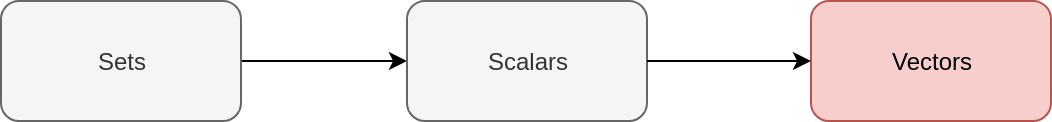# Deep (learning) like Jacques Cousteau - Part 3 - Vectors

(TL;DR: Vectors are ordered lists of numbers.)

LaTeX and MathJax warning for those viewing my feed: please view directly on my website for MathJax goodness!Sector, vector, the lyric inspector
X-Ray vision, power perfector

Kool Keith from ‘MC Champion’ by Ultramagnetic MC’s

Last time, we learnt about scalars. We’ll now start learning about vectors!# Today’s topic: Vectors

## What’s a Vector?

A vector is essentially a list of numbers! They’re normally depicted as columns of numbers (i.e. as column vectors):

The order in which each number appears in our vector is important. For example:

However:

We can transpose vectors so that the rows become columns, or so that the columns become rows. We’ll use an upper case “T” to denote the transpose operator:

We now have ourselves a row vector! Applying the transpose operation on our row vector brings us right back to where we started:

## A tiny bit of notation

We will use the notation from Goodfellow, Ian, et al. and refer to vectors using lower case, bold letters:

The individual elements of our vectors are often called elements, components or entries. We’ll follow Goodfellow, Ian, et al. and call them elements. We’ll refer to the $\boldsymbol{i{\textrm{th}}}$ element of our vector $\boldsymbol{x}$ using an italicised $x$ with a subscript indicating the element number:

For example, we’ll represent the second element of our vector $\boldsymbol{x}$ like this:

## How can we represent vectors in R?

### Common vector types

We have many vector types in R. The vector types that I most commonly use are these:

• numeric,
• character, and
• logical

### Coercing them vectors

In R, all elements of our vectors must be of the same type. If a single element in our vectors violates this condition, the entire vector is coerced into a more generic class. For example, this results in an integer vector:

x <- c(2L, 3L, 5L)
class(x)

##  "integer"


However, this results in a numeric vector:

x <- c(2L, 3L, 5L, 7)
class(x)

##  "numeric"


This makes sense as we learnt in part one that our set of integers, $\mathbb{Z}$, is a subset of our set of real numbers, $\mathbb{R}$. That is:

The $\subset$ symbol means “is a proper/strict subset of”. It indicates that all elements of the set on the left are contained within the set on the right.

This means that we can represent both our integers and real numbers in a single vector of type numeric. However, we can’t represent all of our real numbers in a vector type of integer.

### How can we create vectors?

We can create vectors by using the c() function. For example, here is a numeric vector:

x <- c(1, 2, 3)
print(x)

##  1 2 3


Notice that when we print it out, it looks like a row vector. However, when we use our vector in a data.frame, we get this:

x_df <- data.frame(x)
print(x_df)

##   x
## 1 1
## 2 2
## 3 3


Looks like a column to me! (Note: The numbers in the column on the left are row numbers)

If we then transpose it, we get this:

x_df_transposed <- t(x_df)
print(x_df_transposed)

##   [,1] [,2] [,3]
## x    1    2    3


Looks like a row vector now! Exciting! If we transpose it again, we’re back to a column vector:

t(x_df_transposed)

##      x
## [1,] 1
## [2,] 2
## [3,] 3


# Conclusion

Vectors are ordered lists of numbers. We transposed the hell out of one.

Next time, we’ll combine our knowledge of scalars with our new knowledge of vectors!

Justin

Categories:

Updated: